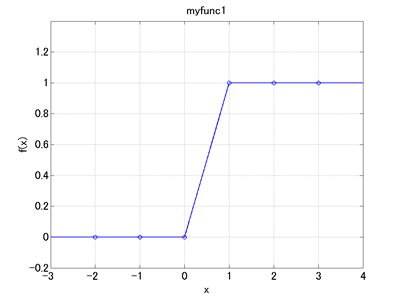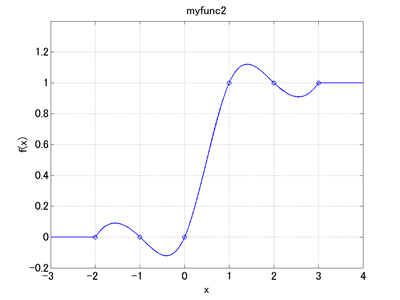Home > Resources > MBDyn Tutorial > 24. Defining and using scalar functions
MBDyn Tutorial

## 24. Defining and using scalar functions

In an input file we can define scalar functions and use them. A scalar function is a function like y = f(x) that relates a real number input (x) with a real number output (y). Effective use of scalar functions will widen the possibility of model creation.

### Defining scalar functions

A scalar function is defined with a "scalar function" card. Definition of a scalar function with a scalar function card can be put anywhere in the input file (even outside of the blocks) as long as it is before the function is called. Below are a couple of examples that define the scalar functions "myfunc1" and "myfunc2" of the "multilinear" and the "cubicspline" types, respectively.

```scalar function: "myfunc1",
multilinear, do not extrapolate,
-2.0, 0.0,
-1.0, 0.0,
0.0, 0.0,
1.0, 1.0,
2.0, 1.0,
3.0, 1.0;
```Figure 1: Graph of the scalar function "myfunc1"

```scalar function: "myfunc2",
cubicspline, do not extrapolate,
-2.0, 0.0,
-1.0, 0.0,
0.0, 0.0,
1.0, 1.0,
2.0, 1.0,
3.0, 1.0;
```Figure 2: Graph of the scalar function "myfunc2"

A way to visualize defined scalar functions as in Figure 1 and 2 is explained in the next chapter.

### Using scalar functions

Once a scalar function is defined we can use it freely in the input file. We can evaluate a scalar function for a specified input as follows.

```model::sf:: <scalar function name> ( <x> )
```

For example, we can use scalar functions when we define a variable as

```set: real A = model::sf::myfunc1(0.5);
```

or when we define a string drive as

```string, "5.e-2*model::sf::myfunc1(Time)";
```

By the way, "Time" is an MBDyn's predefined variable that holds the value of the current simulation time.